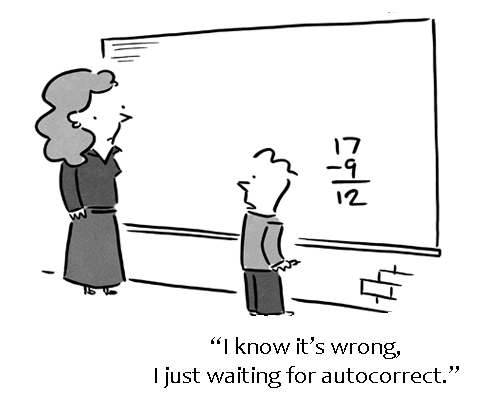Problems
203 - Diffy

Diffy

Time Limit: 1 sec

The Problem

Make a simple program that reads two variables named A, B. Calculate and print the difference of A and B (A - B).The Input

The input file contains 2 integer numbers A and B (A<1000 and B<1000).

The Output

Output will show the difference of A and B in a separate line.

Sample Input

200 50

Sample Output

150

Problem Setter: Shahin ShamS